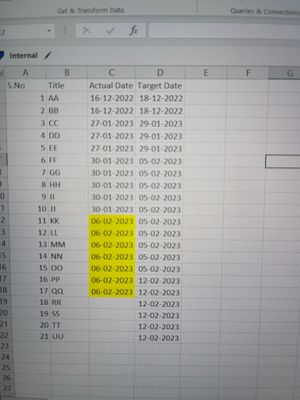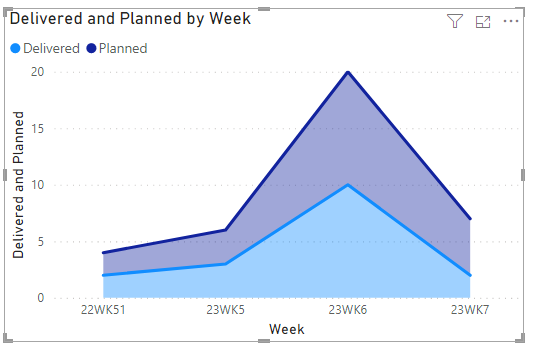cancel
Showing results for
Did you mean:New Member

## Actual vs target Area chart

i have table where each title's actual and completed date.Target date will be the end of the week .Actual date will within or more than the target date.I want to create a area chart showing actual vs target for each week like below1 ACCEPTED SOLUTIONCommunity Support

Hi, @Jameel

You can try the following methods. Create a new date table.

``Week = RIGHT(Year([Date]),2)&"WK"&WEEKNUM([Date],2)``Column:

``Actual Week = IF([Actual Date]<>BLANK(),RIGHT(Year([Actual Date]),2)&"WK"&WEEKNUM([Target Date],2))``
``Target Week = RIGHT(Year([Target Date]),2)&"WK"&WEEKNUM([Target Date],2)``Measure:

``Planned = CALCULATE(COUNT('Table'[S.No]),FILTER(ALL('Table'),[Target Week]=SELECTEDVALUE('Date'[Week])))``
``Delivered = CALCULATE(COUNT('Table'[S.No]),FILTER(ALL('Table'),[Actual Week]=SELECTEDVALUE('Date'[Week])))``Is this the result you expect?

Best Regards,

Community Support Team _Charlotte

If this post helps, then please consider Accept it as the solution to help the other members find it more quickly.

4 REPLIES 4Community Support

Hi, @Jameel

You can try the following methods. Create a new date table.

``Week = RIGHT(Year([Date]),2)&"WK"&WEEKNUM([Date],2)``Column:

``Actual Week = IF([Actual Date]<>BLANK(),RIGHT(Year([Actual Date]),2)&"WK"&WEEKNUM([Target Date],2))``
``Target Week = RIGHT(Year([Target Date]),2)&"WK"&WEEKNUM([Target Date],2)``Measure:

``Planned = CALCULATE(COUNT('Table'[S.No]),FILTER(ALL('Table'),[Target Week]=SELECTEDVALUE('Date'[Week])))``
``Delivered = CALCULATE(COUNT('Table'[S.No]),FILTER(ALL('Table'),[Actual Week]=SELECTEDVALUE('Date'[Week])))``Is this the result you expect?

Best Regards,

Community Support Team _Charlotte

If this post helps, then please consider Accept it as the solution to help the other members find it more quickly.Community Support

Hi, @Jameel

What is the Y-axis in the output you expect?

Best Regards,

Community Support Team _Charlotte

If this post helps, then please consider Accept it as the solution to help the other members find it more quickly.New Member

I want actual and target count on each weekNew Member

If i need to add extra data to the existing data to get the result.please let mw know